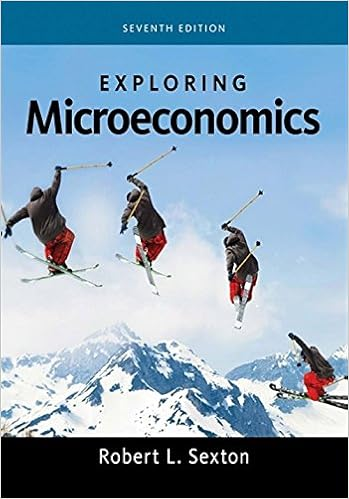# For each of the pair of correlation coefficients provided,...

• Homework Help
• 5
• 93% (14) 13 out of 14 people found this document helpful

This preview shows page 1 - 2 out of 5 pages.

##### We have textbook solutions for you!
The document you are viewing contains questions related to this textbook.The document you are viewing contains questions related to this textbook.
Chapter 1 / Exercise 1
Exploring Economics
SextonExpert Verified
Chapter 13 18) For each of the pair of correlation coefficients provided, determine which one indicates a stronger relation between variables: A] -0.28 and –0.31. The second correlation coefficient (-0.31) represents a stronger negative correlation between variables than the first correlation coefficient (-0.28). B] 0.79 and 0.61. The first correlation coefficient (0.79) represents a stronger positive correlation between variables than the second correlation coefficient (0.61). C] 1.0 and –1.0. According to our textbook, the first correlation coefficient (1.0), which represents a positive correlation, and the second correlation coefficient (-1.0) which represents a negative correlation, are perfect correlations. In other words, neither is stronger than the other. They both are perfect correlations. D] -0.15 and 0.13 The first correlation coefficient (-0.15) represents a stronger negative correlation between variables than the second correlation coefficient (0.13). 22) Calculate degrees of freedom and the critical values, or cutoffs, assuming a two-tailed test with a level of 0.05, for each of the following designs: A] Forty students were recruited for a study about the relation between knowledge regarding academic integrity and values held by students, with the idea that students with less knowledge would care less about the issue than students with greater amounts of knowledge. p
##### We have textbook solutions for you!
The document you are viewing contains questions related to this textbook.The document you are viewing contains questions related to this textbook.
Chapter 1 / Exercise 1
Exploring Economics
SextonExpert Verified
•••